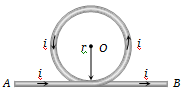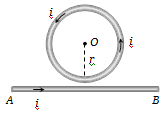NEET Questions Solved

A part of a long wire carrying a current i is bent into a circle of radius r as shown in figure. The net magnetic field at the centre O of the circular loop is(a) $\frac{{\mu }_{0}i}{4r}$                                 (c) $\frac{{\mu }_{0}i}{2\mathrm{\pi r}}\left(\mathrm{\pi }+1\right)$

(b) $\frac{{\mu }_{0}i}{2r}$                                 (d) $\frac{{\mu }_{0}i}{2\mathrm{\pi r}}\left(\mathrm{\pi }-1\right)$

(c) The given circuit can be considered as follows${B}_{loop}=\frac{{\mu }_{0}i}{2r}\odot$

${B}_{conductor}=\frac{{\mu }_{0}i}{2\mathrm{\pi r}}\odot$

${B}_{net}=\frac{{\mu }_{0}i}{2\mathrm{\pi r}}\left(\mathrm{\pi }+1\right)\odot$

Difficulty Level:

• 7%
• 26%
• 55%
• 14%